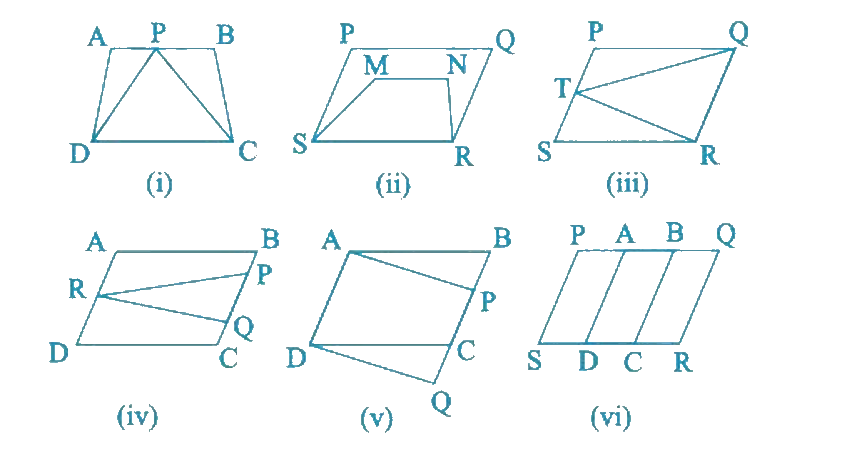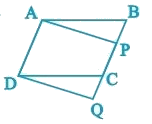# Exercise E9.1 Areas of Parallelograms and Triangles NCERT Solutions Class 9

Go back to  'Areas of Parallelograms and Triangles'

## Chapter 9 Ex.9.1 Question 1

Which of the following figures lie on the same base and between the same parallels. In such a case, write the common base and the two parallels.

### Solution

What is known?

Quadrilaterals consist two or more than two figures.

What is unknown?

Which figures having same base and are they lie between parallel lines or not.

Reasoning:

Same base means length of base must be equal of both figures and touch the line parallel to base.Steps:

(i)Yes, Figure (i) lie on the same base and between the same set of parallel lines.

It can be observed that trapezium $$ABCD$$ and triangle $$PCD$$ have a common base $$CD$$ and these are lying between the same set of parallel lines $$AB$$ and $$CD$$.

(ii)No, Figure (ii) does not satisfy the condition of having a common base and lying between the same set of parallel lines.

It can be observed that parallelogram $$PQRS$$ and trapezium $$MNRS$$ have a common base $$RS$$. However, their vertices, (i.e., opposite to the common base) $$P$$, $$Q$$ of parallelogram and $$M$$, $$N$$ of trapezium, are not lying on the same line. Hence parallelogram $$PQRS$$ and trapezium $$MNRS$$ do not lie between the same set of parallel lines.

(iii)Yes, Figure (iii) lie on the same base and between the same set of parallel lines.

It can be observed that parallelogram $$PQRS$$ and triangle $$TQR$$ have a common base $$QR$$ and they are lying between the same set of parallel lines $$PS$$ and $$QR$$.

(iv)No, Figure (iv) does not satisfy the condition of having a common base and lying between the same set of parallel lines.

It can be observed that parallelogram $$ABCD$$ and triangle $$PQR$$ are lying between the same set of parallel lines $$AD$$ and $$BC$$. However, these do not have any common base.

(v)Yes, Figure (v) lie on the same base and between the same set of parallel lines.

It can be observed that parallelogram $$ABCD$$ and parallelogram $$APQD$$ have a common base $$AD$$ and these are lying between the same set of parallel lines $$AD$$ and $$BQ$$.

(vi)No, Figure (vi) does not satisfy the condition of having a common base and lying between the same set of parallel lines.

It can be observed that parallelogram $$PBCS$$ and $$PQRS$$ are lying on the same base $$PS$$. However, these do not lie between the same set of parallel lines.

Related Sections
Related Sections
Instant doubt clearing with Cuemath Advanced Math Program